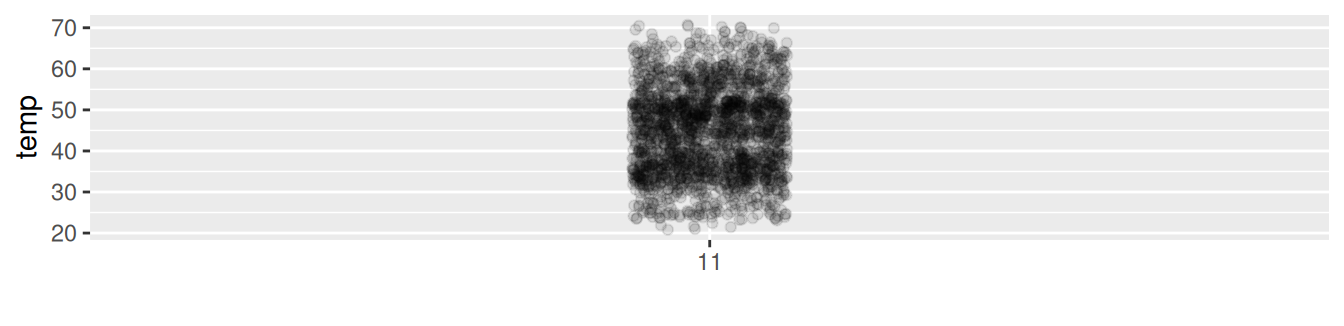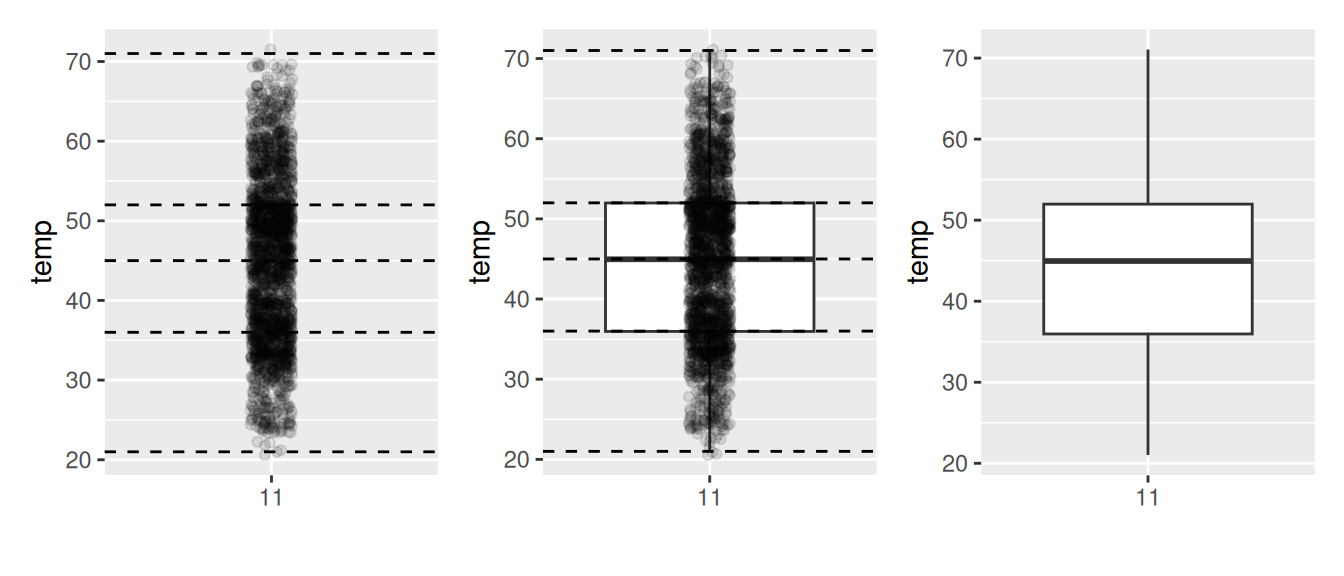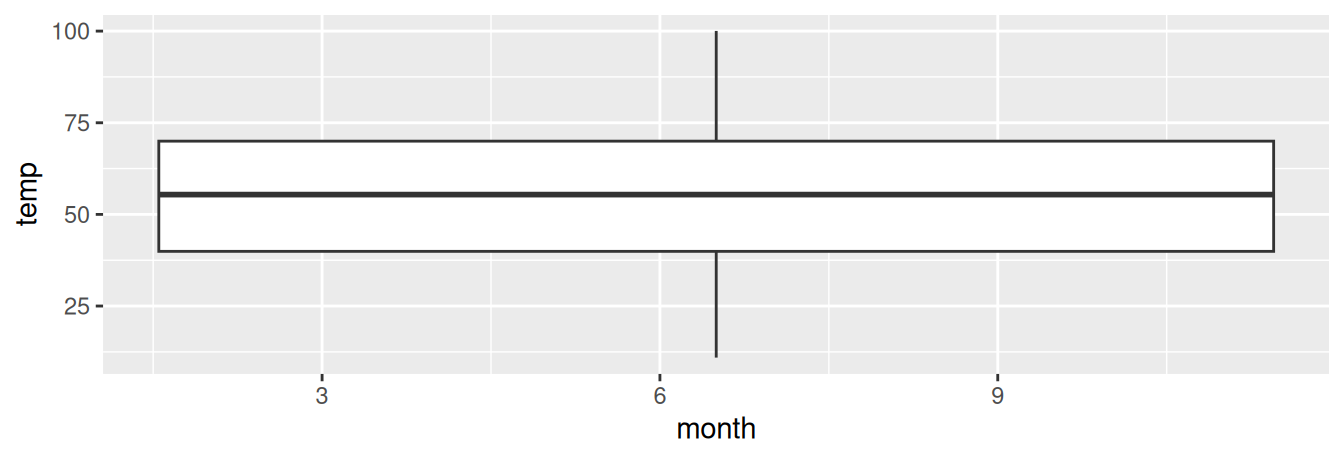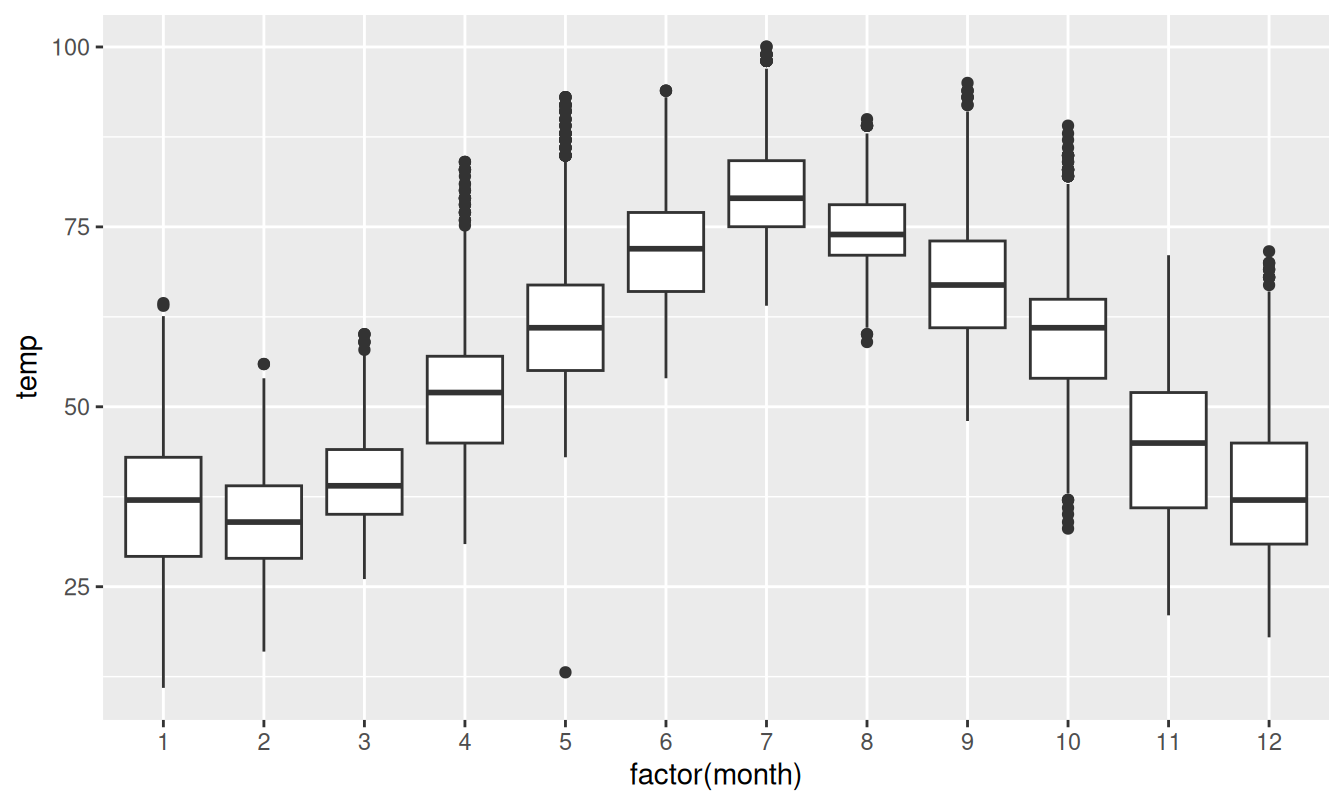## 2.7 5NG#4: Boxplots

While faceted histograms are one type of visualization used to compare the distribution of a numerical variable split by the values of another variable, another type of visualization that achieves this same goal is a side-by-side boxplot. A boxplot is constructed from the information provided in the five-number summary of a numerical variable (see Appendix A.1).

To keep things simple for now, let’s only consider the 2141 hourly temperature recordings for the month of November, each represented as a jittered point in Figure 2.15.FIGURE 2.15: November temperatures represented as jittered points.

These 2141 observations have the following five-number summary:

1. Minimum: 21°F
2. First quartile (25th percentile): 36°F
3. Median (second quartile, 50th percentile): 45°F
4. Third quartile (75th percentile): 52°F
5. Maximum: 71°F

In the leftmost plot of Figure 2.16, let’s mark these 5 values with dashed horizontal lines on top of the 2141 points. In the middle plot of Figure 2.16 let’s add the boxplot. In the rightmost plot of Figure 2.16, let’s remove the points and the dashed horizontal lines for clarity’s sake.FIGURE 2.16: Building up a boxplot of November temperatures.

What the boxplot does is visually summarize the 2141 points by cutting the 2141 temperature recordings into quartiles at the dashed lines, where each quartile contains roughly 2141 $$\div$$ 4 $$\approx$$ 535 observations. Thus

1. 25% of points fall below the bottom edge of the box, which is the first quartile of 36°F. In other words, 25% of observations were below 36°F.
2. 25% of points fall between the bottom edge of the box and the solid middle line, which is the median of 45°F. Thus, 25% of observations were between 36°F and 45°F and 50% of observations were below 45°F.
3. 25% of points fall between the solid middle line and the top edge of the box, which is the third quartile of 52°F. It follows that 25% of observations were between 45°F and 52°F and 75% of observations were below 52°F.
4. 25% of points fall above the top edge of the box. In other words, 25% of observations were above 52°F.
5. The middle 50% of points lie within the interquartile range (IQR) between the first and third quartile. Thus, the IQR for this example is 52 - 36 = 16°F. The interquartile range is a measure of a numerical variable’s spread.

Furthermore, in the rightmost plot of Figure 2.16, we see the whiskers of the boxplot. The whiskers stick out from either end of the box all the way to the minimum and maximum observed temperatures of 21°F and 71°F, respectively. However, the whiskers don’t always extend to the smallest and largest observed values as they do here. They in fact extend no more than 1.5 $$\times$$ the interquartile range from either end of the box. In this case of the November temperatures, no more than 1.5 $$\times$$ 16°F = 24°F from either end of the box. Any observed values outside this range get marked with points called outliers, which we’ll see in the next section.

### 2.7.1 Boxplots via geom_boxplot

Let’s now create a side-by-side boxplot of hourly temperatures split by the 12 months as we did previously with the faceted histograms. We do this by mapping the month variable to the x-position aesthetic, the temp variable to the y-position aesthetic, and by adding a geom_boxplot() layer:

ggplot(data = weather, mapping = aes(x = month, y = temp)) +
geom_boxplot()FIGURE 2.17: Invalid boxplot specification.

Warning messages:
1: Continuous x aesthetic -- did you forget aes(group=...)?
2: Removed 1 rows containing non-finite values (stat_boxplot). 

Observe in Figure 2.17 that this plot does not provide information about temperature separated by month. The first warning message clues us in as to why. It is telling us that we have a “continuous”, or numerical variable, on the x-position aesthetic. Boxplots, however, require a categorical variable to be mapped to the x-position aesthetic. The second warning message is identical to the warning message when plotting a histogram of hourly temperatures: that one of the values was recorded as NA missing.

We can convert the numerical variable month into a factor categorical variable by using the factor() function. So after applying factor(month), month goes from having numerical values just the 1, 2, …, and 12 to having an associated ordering. With this ordering, ggplot() now knows how to work with this variable to produce the needed plot.

ggplot(data = weather, mapping = aes(x = factor(month), y = temp)) +
geom_boxplot()FIGURE 2.18: Side-by-side boxplot of temperature split by month.

The resulting Figure 2.18 shows 12 separate “box and whiskers” plots similar to the rightmost plot of Figure 2.16 of only November temperatures. Thus the different boxplots are shown “side-by-side.”

• The “box” portions of the visualization represent the 1st quartile, the median (the 2nd quartile), and the 3rd quartile.
• The height of each box (the value of the 3rd quartile minus the value of the 1st quartile) is the interquartile range (IQR). It is a measure of the spread of the middle 50% of values, with longer boxes indicating more variability.
• The “whisker” portions of these plots extend out from the bottoms and tops of the boxes and represent points less than the 25th percentile and greater than the 75th percentiles, respectively. They’re set to extend out no more than $$1.5 \times IQR$$ units away from either end of the boxes. We say “no more than” because the ends of the whiskers have to correspond to observed temperatures. The length of these whiskers show how the data outside the middle 50% of values vary, with longer whiskers indicating more variability.
• The dots representing values falling outside the whiskers are called outliers. These can be thought of as anomalous (“out-of-the-ordinary”) values.

It is important to keep in mind that the definition of an outlier is somewhat arbitrary and not absolute. In this case, they are defined by the length of the whiskers, which are no more than $$1.5 \times IQR$$ units long for each boxplot. Looking at this side-by-side plot we can see, as expected, that summer months (6 through 8) have higher median temperatures as evidenced by the higher solid lines in the middle of the boxes. We can easily compare temperatures across months by drawing imaginary horizontal lines across the plot. Furthermore, the heights of the 12 boxes as quantified by the interquartile ranges are informative too; they tell us about variability, or spread, of temperatures recorded in a given month.

Learning check

(LC2.22) What does the dot at the bottom of the plot for May correspond to? Explain what might have occurred in May to produce this point.

(LC2.23) Which months have the highest variability in temperature? What reasons can you give for this?

(LC2.24) We looked at the distribution of the numerical variable temp split by the numerical variable month that we converted using the factor() function in order to make a side-by-side boxplot. Why would a boxplot of temp split by the numerical variable pressure similarly converted to a categorical variable using the factor() not be informative?

(LC2.25) Boxplots provide a simple way to identify outliers. Why may outliers be easier to identify when looking at a boxplot instead of a faceted histogram?

### 2.7.2 Summary

Side-by-side boxplots provide us with a way to compare the distribution of a numerical variable across multiple values of another variable. One can see where the median falls across the different groups by comparing the solid lines in the center of the boxes.

To study the spread of a numerical variable within one of the boxes, look at both the length of the box and also how far the whiskers extend from either end of the box. Outliers are even more easily identified when looking at a boxplot than when looking at a histogram as they are marked with distinct points.